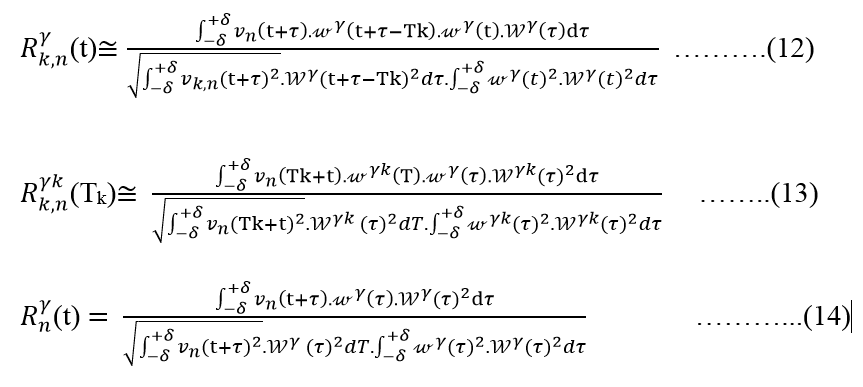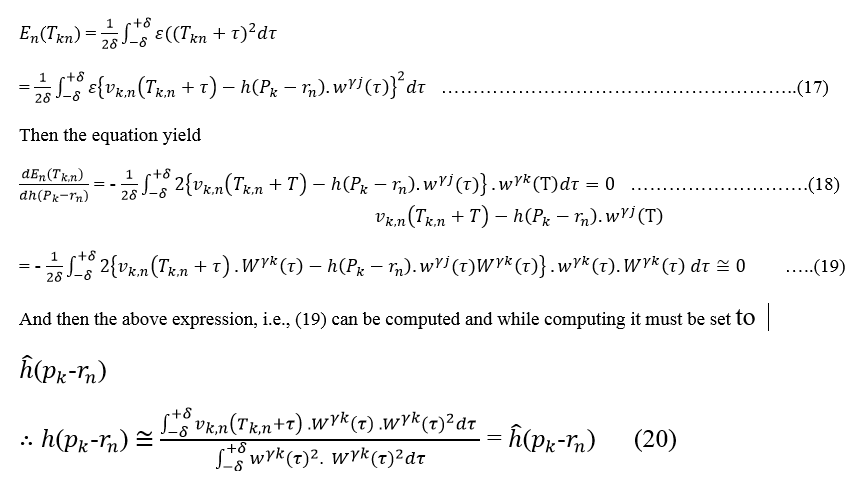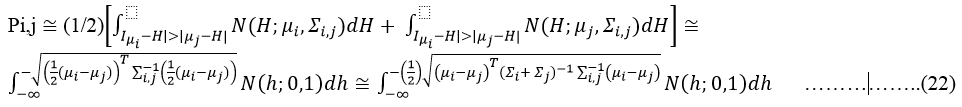Raju: Investigation of multi-site micro recordings of subthalamic nucleus neurons using machine learning MER with DBS in Parkinson`s – A simulation study

Introduction

Separation of action potentials (spikes) generated by adjacent neurons is a major problem in extracellular microelectrode recording from cell-dense regions of the brain (e.g., hippocampal pyramidal cell layer). Although several types of hardware/software window discrimination have been devised, the number of neurons separable from single-channel recordings is small, averaging about two.1 Conventional procedures cannot distinguish between similar-amplitude spikes originating from different neurons equidistant from the recording microelectrode. Part of the problem has been solved by using the stereotrode method,2 which discriminates between several neurons based on the peak-amplitude ratio of spikes detected at two adjacent electrodes. Peak amplitude, however, is only one spike feature, and is unstable at low signal-to-noise (S/N) ratio or low sampling rates. Recently, a neural network method was applied to stereotrode data for multineuronal classification.3 When new multichannel spike data are to be clustered, this method requires the result of prior clustering of spike data obtained under identical conditions (the same electrode, multineuronal positions, etc.). Such a requirement imposes limitations on the applicability of the method to a variety of multichannel spike data.

We propose here a substantial improvement of the stereotrode method by using covariance, rather than just the peak-amplitude ratio, between a template and an actual spike recorded using a multisite microelectrode. Partially overlapping spikes a major obstacle to spike discrimination were separated by successively subtracting preceding spikes from raw data. Another obstacle complex spike bursts, i.e., series of spikes with different amplitudes was overcome by first detecting such bursts and then using only the first spike of each burst for spike clustering. These improvements produced better spike cluster separation. We also confirmed the correspondence between single clusters and neurons using the neurophysiological collision test. 4

Objectives

To investigate the multiunit multisite microelectrode recordings of subthalamic nucleus neurons with deep brain stimulation in Parkinson`s by applying the machine learning methods, signal, and cluster analysis.

Materials and Methods

Conventional window discriminators separate neuronal spikes by mainly referencing their peak amplitudes. However, boundaries of spike amplitudes between different neurons are difficult to determine, e.g., two neurons equidistant from the electrode site may have nearly identical amplitudes. McNaughton et al. developed the stereotrode method in which neuronal spikes are separated by referring to the joint distribution of peak-amplitude values obtained by multichannel recording.2 The method given is based on the same strategy but involves calculation of damping vectors for each observed spike. It also involves automatic decomposition of spikes that are noisy, bursty, or overlapping.

Notion

Action potentials of the target neuron are recorded extra-cellularly with a multisite microelectrode. The action potentials are assumed to originate from the center of the cell body and to exhibit consistent amplitudes at a certain distance from an active electrode site. We normalize the averaged spike waveform so that its negative peak equals 1μV and refer to this normalized spike waveform ωt as a template.

A spike generated at time T by the target neuron located at P is described as where htp-rn is the impulse response function of the damping factor (i.e., volume conduction from the target neuron to the nth electrode site), t indicates time, and is the convolution operator. Consider a spike computed at the duration of time T via the target neuron situated at a point p is defined as

vn(t)=ht, p-rn×ωt-T ........(1)

where htp-rn is the impulse response function of the damping factor, i.e., volume conduction from the target neuron

to the nth electrode site, t indicates time, and is the convolution operator. By focusing on the attenuation effect of the spatial damping factor, (1) is transformed as

By focusing on the attenuation effect of the spatial damping factor, (1) is transformed as

vn(t)=h(p-rn). ω(t-T)+εnt  ......(2)

By minimization of the energy of the error function, is estimated as hp-rn and then

vn(t)h(p-rn). ω(t-T)  .......(3)

Since ωt is normalized so that its negative peak equals

1μVhp-rn approximately equals the negative peak amplitude of each spike. The spatial damping factors from multisite electrode N points are computed and vectorized as follows

H^p=h^(p-r1,h^(p-r2,h^(p-r3,....h^(p-rN

.....(4)

If the point of the electrode is fixed, the spatial dampening-vector H^(p) relates to the location of the neural-target, i.e., target-neuron, ‘p’. Therefore, spatial damping vectors of spikes generated by the target neuron form a cluster in damping vector space and clusters of damping vectors correspond to different neurons. Spatial damping vectors are clustered by hierarchical clustering incorporating a multidimensional statistical test with the null hypothesis the distributions of two clusters are identical.

Pliable stretchy template reflects elastic

In template matching for detecting neuronal spikes,5 template waveforms should be selected, considering the variety of observed spike waveforms. Spike waveforms generated by neurons in the vicinity of a microelectrode are similar in that each contains a negative peak and a subsequent positive peak. However, spike amplitude and duration vary among different neurons.6 The differences in spike amplitude are used to determine which neuron generated a spike. Differences in spike duration make spike detection using a fixed template difficult, so we propose using an elastic template that can be fitted to different spike durations.

In preliminary spike detection based on template matching, a large spike is arbitrarily chosen as a template waveform. Spike waveforms detected in this way are then averaged. The averaged waveform is normalized so that its negative peak equals 1μV and used as the original template ψt Based on the original template ψt elastic template ψyt

is defined as wγ=ψty .....(5)

where Y indicates the duration ratio of ωy(t) to ψ(t) i.e., the resistance of ψ(t) is described by Y The optimum value of is determined so that each spike detected has a maximum correlation with ωy(t) at optimized Y When elastic template ωy(t) is used instead of ω(t) in the previous subsection, undesirable effects of variable spike durations are minimized in calculating spike damping vectors.

Identifying the spikes

The correlation method has been widely used for detecting neuronal spikes. In this method recorded signals having high correlation coefficients with a template are recognized as spikes.5 Detection of superimposed spikes generated by multiple neurons is a major challenge for any spike detection method. Several methods have been devised to detect such superimposed spikes. The most frequently used method is called the exhaustive search technique in which all possible combinations of predetermined templates are time-shifted to search for the best template match.7, 8 The main prob-lem of this method is the large amount of computational time required to process all possible combinations of time-shifted templates. We modify the correlation method, which is less time-consuming than the method above, such that superimposed spikes are separated by the subtraction technique described in Section II-C2.

The correlation coefficient between the observed waveform for each channel viti=1 To N and elastic template of wγ(t) the spike detector is compared with threshold (Th). If one of the correlation coefficients exceeds Th, a spike is considered to have been detected.

Computing the correlation-coefficient

In order to improve the identification of the signal-spikes, the weighting-vector function can be applied for computing the correlation-coefficients. Hence, by utilizing the weight-function, the analyzed signal at the n-th electrode point wn(t) contains corresponding coincide and interrelating-spikes

vn=j=-+vj,  n(t)   .......(6)

Where-in, vj,  nt giving the j-th analyzed spike produced by any neural-cell within the area of the n-th electrode-point at Ti time. Therefore, presuming that the weight-function Wγ(t) weaken the spikes occurring before and after the spike observed at , with spike duration ratio . Here, we used a smoothed square waveform of the elastic template for weighting

wyt=-δ8+δ8wγ(t+τ)2dτ-δ8+δ8wγ(τ)2dτ   ................(7)

i.e.,

wy(t)=-δ8+δ8wγt2+τ2+2tτdτ-δ8+δ8wγ(τ)2dτ .........(8)

Where δ indicates the duration of the spike train which is 1±3 milliseconds. (±1 m Sec to ±3 m Sec). By using this weight vector letting us to isolate the neural spike examined at Tk as given in the following equation (9)

vk,ntvnt. wyt-Tk.......(9)

Therefore, can convert the pattern-signal template as

wγtwyt. wyt-Tk......(10

Now we can compute the correlation-coefficient between vk,n(t) and the template (i.e., the variable-template) as

Rk,ny(t)=-δ+δvk,nt+τ.wγ(τ)dτ-δ+δ vk,n(t+τ)2dT.-δ+δwγ(τ)2dτ....(11)

Therefore, we can convert the equation (11) to equation (12) by applying the equation (9), as shown in below expression

Figure 0Because Rk,nγ(t) is maximal when the k-th neural-spike occur, i.e., t=Tk, and the ratio of time-duration γk, we can identify any spike by verifying Rnγt. Or else, every spike is identified as every time-period when Rnγt of any n (n=1,2,3,…N) and any γ which is equivalent ( γ= γ min to γmax, and exceeds the Th determined in advance.

2) Separation of Preceding Spikes: If the th spike and those preceding it overlap, the positive deflection of preceding spikes causes errors in estimation of damping factor h(pk-rn)(n=1 to N)

To minimize such errors, we subtract the components of preceding spikes from observed waveform

Rk,nγk(Tk)Rkγk(Tk)     .....(15)

vnkt=jk+vj,n(t) =vnt-j=-k=1vj,n(t)vn(t)-jk+h^(pj-rn). wyj(t-Tj,n)  .....(16)

By connecting the vn(k)(t) as vn(t) in equation (14), the k-th neural-spike is characterized or distinguished from vn(k)(t) unaltered through positive (+Ve) deflections or refractions of previous train neural-spikes.

Computing the damping factorial

The turning points of the spike-train that are peaks acquired at various micro electrode points might have small and insignificant but then constant setbacks in some situations as multiunit data sampling synchronously (not concurrently or parallel). Say, even if the correlation function for the k-th spike observed at the i-th electrode point has a local peak value of Riγk(Tk,j) at time Tk,i, that observed at the j-th site may not have a local peak at time Tk,i, but have a local peak of Rjγk(Tk,j) at time Tk,j, Hence, the timing of spike appearance at each point is adjusted and evaluated again during the period when Rnγ(t) of any n (n=1 to N) and any gamma (gamma = gamma min to gamma max) is over Th. After timing adjustment, damping factor h(pk-rn) is estimated by minimizing the mean-square-error (MSE) en(t) near the time Tk,n, namely, by applying the estimation function

Figure 0Hence, it is set to h^pk-rn

Now, if -δ+δwγk(τ)2dτ=1, the expression (20) is identically equal to the covariance between vnTk,n+t and also wγkτ. If h^pk-rn of the k-th spike of the STN potential train is lower than the noise-distortion ρ, we neglect such a small spike after computing the damping factorials electrode points, i.e., sites, we neglect such an iota of train-spike after computing the curbing (or dampening) factors.

Managing burst action potential train spikes

Most subthalamic nucleus neuron jones are detected and microelectrode recording with bursting patterns can identify subthalamic neurons by their characteristic bursting pattern and their signals clearly identify the STN nucleus neurons form the surrounding structures with decreasing amplitudes, i.e., complex bursts of the spikes.9 Smaller spikes of such a complex spike burst tend to be misclassified as having been generated by different neurons. We describe here a procedure to avoid such misclassification. Since the first spike of a spike burst is considered not affected by the firing mode, the damping vectors of subsequent spikes are represented by the first spike. The subsequent spikes are then classified as belonging to the cluster of the first spike. A pair of spikes is considered to occur in a burst under the following conditions: the inter-spike-interval (ISI) is ISI b_min, ISI bax),. tTypical firing pattern with irregular firing and broad baseline noise is noticed from -1.00 level onwards…

So, they are known to fire a burst of train-spikes with reducing amplitudes, i.e., complex spike bursts

Cluster analysis

The spatial dampening vectors of the train-spikes computed/ or generated by a STN neuron are homo-centrically as a cluster in a multi-dimensional space

… cluster is characterized or embodied as a normal distribution of NH;μii:

NH;μii=12π2Σie-12H-μiTΣi-1(H-μi) (21)

Wherein ‘H’ indicating the dampening-vector as a static, ‘N’ is the height of the multi-dimensional-space equivalent to the number of electrode points, followed by the μi, Σiare mean of population plus variance and covariances of dampening-vectors included in the i-thcluster.

The spatial damping vectors are clustered hierarchically by the centroid method.10 For the clustering algorithm, a Mahalanobis generalized distance is defined as the distance between the i-th and j-th clusters. The Mahalanobis generalized distance is concerned with the error rate in which damping vectors in the i-th cluster are misclassified into the j-th cluster and vice versa, i.e., the rate of the first and second type errors. 11

We have two assumptions; (1) spikes in the i-th cluster occur at the same rate as those in the j-th cluster, (2) the population variance-covariance matrix of the damping vectors included in the i-th cluster is nearly equal to that of damping vectors in the j-th cluster, i.e.,

ij1-i+j=ij

And we can compute the error-rate such as

Figure 0Here, H is the simplified Mahalananobis distance (statistic domain). If maximum of Pij=PIJ among all set groups (or of clusters) then i-th and j-th clusters are merged and the cluster analysis is resumed as long is PIJ 0.016, i.e., The simplified distance of Mahalananobis

μi-μjT12(ΣI+ ΣJ)-14

Therefore, the terminal constraint of the cluster-process is determined by the Mahalananobis statistical test. Since  μi Σiare unknown until the end of the clustering, substitute values

Since and are unknown until the end of clustering,

Substitute values pq used. Since each damping vector initially forms a cluster, the initial value of μi^ is the i th damping vector. Since cluster distribution is scattered due to noise or small overlapping spikes, the diagonal elements   i of are replaced by the variance of noise or background spikes, σ2. The other elements are set to zero when the number of damping vectors included in the i th cluster is less than N. If the number of damping vectors included in the ith cluster is greater than N after a few clustering iterations, nondiagonal elements of i are set to λσ2, where λ is the correlation coefficient between the pth and qth components of the damping vectors in the i-th cluster.

Results and Discussion

In this study, we understood the equivalence of a cluster-group with a subthalamic nucleus neuron. However, the validity of this notion has not yet been determined. We accomplished further investigations in which an aimed-target s t n neuron was detected by the collision-test and its damping vectors were determined by our clustering method. Since the damping vectors of the detected neuron distilled in a cluster-group which communicated well to one of the cluster-groups in the multi cluster-group allocation, demonstrating the neuron cluster correspondence. Stimulus-amplitude and pulse-widths of window discriminators of stn-neural-spikes acquired with a single site single unit microelectrode is equivalent to cluster-groups of neural-spikes including source to a minimal-distribution of damping-vectors. In distinction with single-site microelectrode recording (MER) signals of STNs, our cluster-analysis disconnected neuronal-spikes, in particular, by a joint-distribution of damping-vectors. Whether we can achieve any gain from multi-site signal-recording, nevertheless, varies on the form of multi-site multi-unit micro-electrode and on neuron-density. Neuronal-spikes computed or simulated or generated by a neuron in a cell-sparse-region, for instance, are simply separated by single-site micro-electrode recording MER signals of stn. A multi-site multi-unit microelectrode together with numerous adjoining recording-sites is necessary to distinguish neuronal-spikes computed generated/simulated by means of a number of neurons in a cell-dense-region-areas for instance the hippo-campus(campal)/pyramidal cell-layer of rascal`s. Hence, the connection among the form of multi-site multi-unit micro electrode and cell-density should be explored further than to achieve the highest advantage of use of multi-site multi-unit micro-electrode recording (MER) signals of subthalamic and hippocampal regions of the non-primate rascal animals.

Conclusion

We developed here a procedure for spontaneously categorizing several neuronal-spikes from multi-site multi-micro-electrode signals recording of stn data. The technique is vigorous sturdy and robust as compared to lower-sampling frequency and noise-distortion because a neuronal-spike is characterized as a spatial-damping-vector derived on based on the covariance-matrix amongst a pattern-matching template and the experimental signal at every single signal-recording-point of contact. The correlation among a cluster-group of damping vectors and a neuron was corroborated by the collision-test. It may be concluded that the developed procedure is useful for further accurate categorization of neuro-nal-spikes than other kinds-of-spike-sorting, which lack the techniques to distinguish corresponding-spikes or to treat spike-bursts. This developed procedure is effective in researching multi neuronal connections in locally connected neuronal-net-works.

Conflict of Interest

The author declares no potential conflicts of interest with respect to research, authorship, and/or publication of this article.

None.

References

1

K H Park Clinical outcome prediction from analysis of microelectrode recordings using deep learning in subthalamic deep brain stimulation for Parkinson‘s diseasePlos One2021161e0244133 10.1371/journal.pone.0244133

2

Koirala Acquisition of MER Data of STN neurons with deep brain stimulation in Parkinson`sScientific Rep20201019241113

3

F Agnesi AT Connolly KB Baker JL Vitek MD Johnson Deep brain stimulation imposes complex informational lesionsPLoS ONE2013268e7446210.1371/journal.pone.0074462

4

M T Barbe T A Dembek J Becker J Raethjen M Hartinger IG Meister Individualized current-shaping reduces DBS-induced dysarthria in patients with essential tremorNeurology2014827614910.1212/WNL.0000000000000127

5

M T Barbe Multiple source current steering-a novel deep brain stimulation concept for customized programming in a Parkinson’s disease patientPark Relat Disord2014204713

6

CK Berenstein L H M Mens J J S Mulder F J Vanpoucke Current steering and current focusing in cochlear implants: comparison of monopolar, tripolar, and virtual channel electrode configurationsEar Hear20082922506010.1097/aud.0b013e3181645336

7

P Blomstedt U Sandvik S Tisch Deep brain stimulation in the posterior subthalamic area in the treatment of essential tremorMov Disord201625101350610.1002/mds.22758

8

B H Bonham LM Litvak Current focusing and steering: Modeling, physiology, and psychophysicsHear Res20082421-21415310.1016/j.heares.2008.03.006

9

L J Bour M A J Lourens R Verhagen R M A de Bie P van den Munckhof P R Schuurman Directional recording of subthalamic spectral power densities in Parkinson’s disease and the effect of steering deep brain stimulationBrain Stimul2015847304110.1016/j.brs.2015.02.00

10

L Breiman Random ForestsMach Learn20014553210.1023/A:1010933404324

11

J Buhlmann Modeling of a segmented electrode for desynchronizing deep brain stimulationFront Neuroeng20114150.3389/fneng.2011.00015

© This is an open access article distributed under the terms of the Creative Commons Attribution-NonCommercial-ShareAlike 4.0 International License which permits unrestricted use, distribution, and reproduction in any medium, provided the original author and source are credited.

Article History

Accepted : 12-12-2021

Available online : 05-01-2022

View Article

Get article permission for commercial use

Viewed: 61